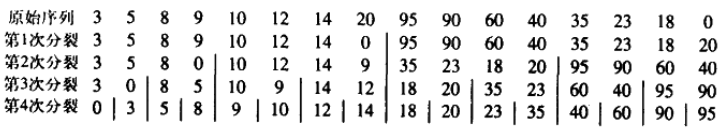# Python之双调排序

17 篇文章 2 订阅

(1)存在一个ak(1≤k≤n), 使得a1≥…≥ak≤…≤an成立；或者
(2)序列能够循环移位满足条件(1)

Batcher定理

Bitonic merge（双调合并）Bitonic Sort（双调排序）

def compAndSwap(a, i, j, dire):
if (dire == 1 and a[i] > a[j]) or (dire == 0 and a[i] < a[j]):
a[i], a[j] = a[j], a[i]

def bitonicMerge(a, low, cnt, dire):
if cnt > 1:
k = int(cnt / 2)
for i in range(low, low + k):
compAndSwap(a, i, i + k, dire)
bitonicMerge(a, low, k, dire)
bitonicMerge(a, low + k, k, dire)

def bitonicSort(a, low, cnt, dire):
if cnt > 1:
k = int(cnt / 2)
bitonicSort(a, low, k, 1)
bitonicSort(a, low + k, k, 0)
bitonicMerge(a, low, cnt, dire)

def sort(a, N, up):
bitonicSort(a, 0, N, up)

if __name__ == "__main__":
# Driver code to test above
a = []

n = int(input().strip())
for i in range(n):
a.append(int(input().strip()))
up = 1

sort(a, n, up)
print("\n\nSorted array is")
for i in range(n):
print("%d" % a[i])

04-27261
12-0724407-31105
12-17670
08-111198
07-131150
02-271827
10-095122
11-2017
11-2038
07-284769
08-0456
08-2826
03-2238
12-25563
03-26365
01-216788
01-09351
11-154万+点击重新获取扫码支付余额充值